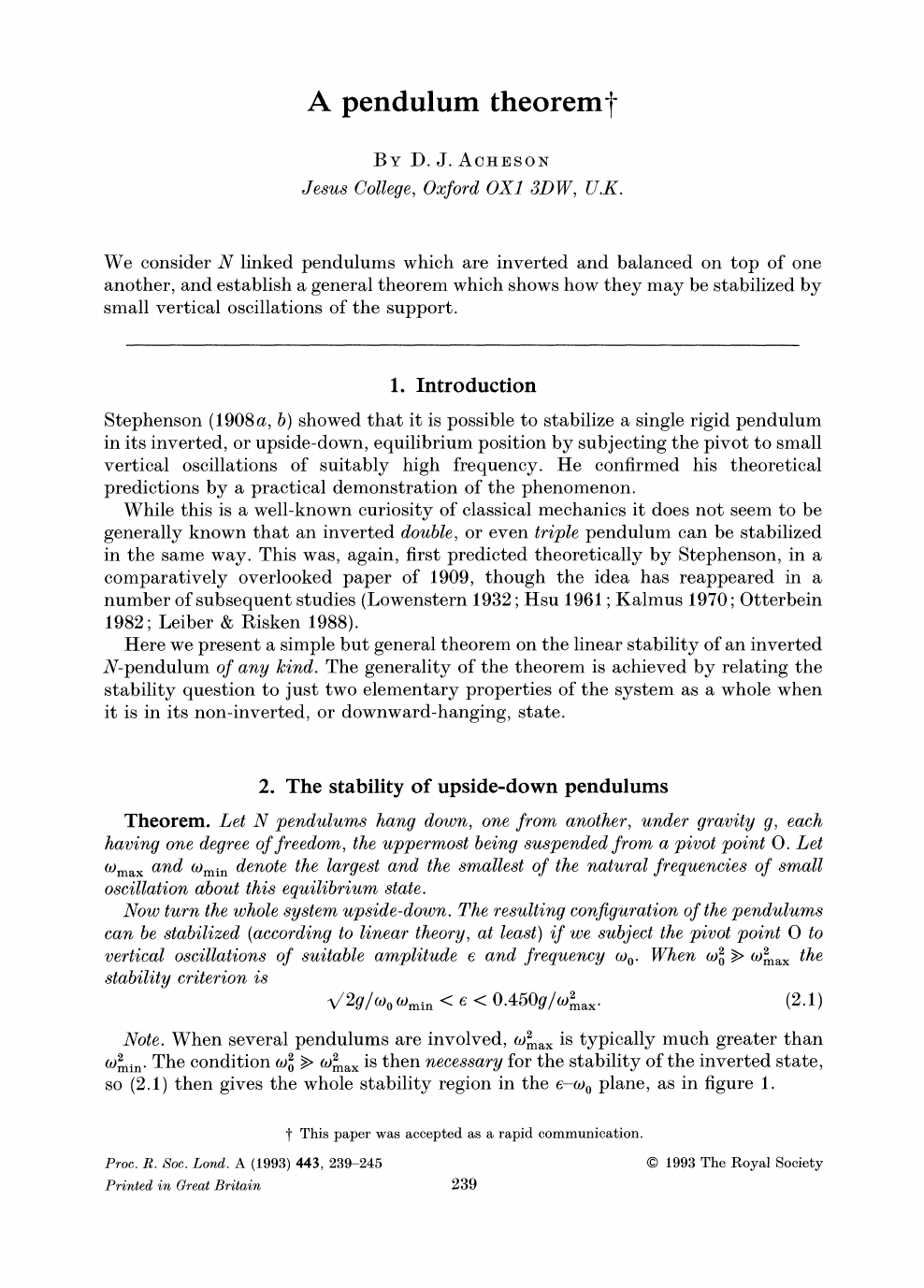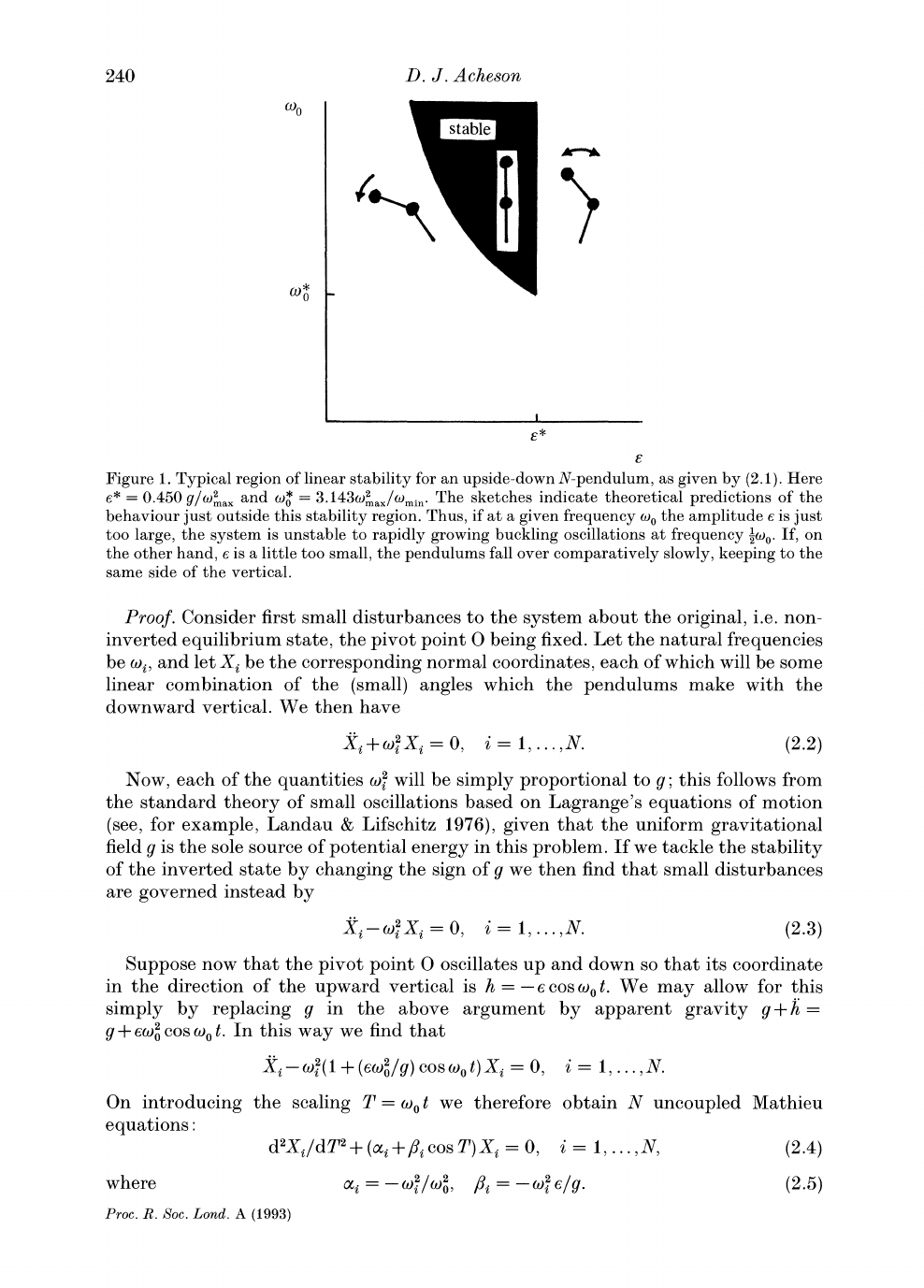This paper was written by D.J. Acheson while he was trying to give ...
In 1908 Stephenson found that the upper vertical position of the pe...
This paper is from 1993! I had thought it was much older on my firs...
As we can see in this video by Steve Mould the chain of pendulums c...
To prove this we are going to use the Lagrangian formulation and wi...
To prove that the natural frequencies are proportional to $g$ we st...
Near $\alpha = 0$, $\sqrt{-2\alpha} <\beta <0.450$. Since $\alpha_... Also the larger the value of$N$, the smaller the value of$l\$ beca...A
pendulum
theoremt
BY D.
J.
ACHESON
Jesus
College,
Oxford
OX1
3DW,
U.K.
We
consider N
pendulums
which are inverted and
balanced
on
top
of
one
another,
and
establish
a
general
theorem which shows
how
they may
be stabilized
by
small vertical
oscillations
of the
support.
1.
Introduction
Stephenson
(1908a, b)
showed
that it
is
possible
to
stabilize
a
single rigid
pendulum
in
its
inverted,
or
upside-down, equilibrium position by
subjecting
the
pivot
to small
vertical oscillations
of
suitably
high frequency.
He confirmed his
theoretical
predictions by
a
practical
demonstration of
the
phenomenon.
While
this
is a well-known
curiosity
of classical mechanics it does not seem to
be
generally
known
that an inverted
double,
or
even
triple
pendulum
can be stabilized
in
the same
way.
This
was,
again,
first
predicted theoretically by Stephenson,
in a
comparatively
overlooked
paper
of
1909,
though
the idea has
reappeared
in a
number of
subsequent
studies
(Lowenstern
1932;
Hsu
1961;
Kalmus
1970;
Otterbein
1982;
Leiber
&
Risken
1988).
Here we
present
a
simple
but
general
theorem on the linear
stability
of an
inverted
N-pendulum
of
any
kind.
The
generality
of the theorem is achieved
by
relating
the
stability
question
to
just
two
elementary
properties
of the
system
as
a
whole when
it
is
in
its
non-inverted,
or
downward-hanging,
state.
2.
The
stability
of
upside-down
pendulums
Theorem.
Let
N
pendulums
hang
down,
one
from
another,
under
gravity
g,
each
having
one
degree
of
freedom,
the
uppermost
being
suspended
from
a
pivot point
O.
Let
O)max
and
wmin
denote
the
largest
and the smallest
of
the natural
frequencies
of
small
this
equilibrium
state.
Now
turn the whole
system
upside-down.
The
resulting configuration
of
the
pendulums
can be stabilized
(according
to linear
theory,
at
least)
if
we
subject
the
pivot point
0
to
vertical
oscillations
of
suitable
amplitude
e
and
frequency
(o.
When
(O2
>
o)ax
the
stability
criterion
is
/V2g/oo
tmin
< e
<
0.450g/2ma.
(2.1)
Note.
When
several
pendulums
are
involved,
T2ax
is
typically
much
greater
than
2i.
The condition
o2
>
Omax
is
then
necessary
for the
stability
of the inverted
state,
so
(2.1)
then
gives
the
whole
stability region
in the
e-o)0
plane,
as
in
figure
1.
t
This
paper
was
accepted
as
a
rapid
communication.
Proc. R. Soc.
Lond. A
(1993)
443,
239-245
?
1993
The
Royal
Society
Printed in Great Britain
239
This content downloaded from 169.229.32.136 on Wed, 7 May 2014 15:58:31 PM
All use subject to JSTOR Terms and Conditions8*
?
Figure
1.
Typical region
of linear
stability
for an
upside-down
N-pendulum,
as
given
by
(2.1).
Here
*
=
0.450
g/Oax
and
co
=
3.1432
ax/(min.
The
sketches
indicate theoretical
predictions
of the
behaviour
just
outside this
stability region.
Thus,
if
at
a
given frequency
w
the
amplitude
e
is
just
too
large,
the
system
is unstable to
rapidly growing buckling
oscillations
at
frequency
-o0.
If,
on
the
other
hand,
e is
a
little too
small,
the
pendulums
fall over
comparatively slowly,
keeping
to the
same side
of
the vertical.
Proof.
Consider
first small
disturbances
to the
system
the
original,
i.e. non-
inverted
equilibrium
state,
the
pivot point
0
being
fixed. Let the
natural
frequencies
be
oi,
and let
Xi
be the
corresponding
normal
coordinates,
each of
which will
be some
linear
combination
of
the
(small)
angles
which the
pendulums
make
with
the
downward vertical.
We
then
have
Xi+?
Xi,=0,
i=l,...,N.
(2.2)
Now,
each of
the
quantities
()2
will be
simply
proportional
to
g;
this
follows from
the standard
theory
of small oscillations based on
Lagrange's
equations
of motion
(see,
for
example,
Landau
&
Lifschitz
1976),
given
that the uniform
gravitational
field
g
is
the
sole
source of
potential
energy
in this
problem.
If
we tackle the
stability
of the inverted state
by changing
the
sign
of
g
we then find that small
disturbances
are
governed
by
Xi-o
Xi
i=0,
i==l,...,N.
(2.3)
Suppose
now that the
pivot point
0 oscillates
up
and
down so that its
coordinate
in
the direction
of
the
upward
vertical
is h
=
-ecoso0t.
We
may
allow for
this
simply by
replacing
g
in the
above
argument by apparent
gravity
g
+h
=
g
+
e()
cos
o0
t.
In this
way
we
find that
X?--0~(?
1
+
(eo)/g)
coS
ot)Xi
=
0,
i
=
1,...,N.
On
introducing
the
scaling
T
=
(ot
we
therefore obtain N
uncoupled
Mathieu
equations:
d2Xi/dT2
+
(ci
+
ficosT)Xi
=
0,
i
=
1,...,N,
(2.4)
where
i
=-2/o2,
/=-i
=-
/g. (2.5)
Proc.
R.
Soc. Lond.
A
(1993)
D. J. Acheson
240
This content downloaded from 169.229.32.136 on Wed, 7 May 2014 15:58:31 PM
All use subject to JSTOR Terms and Conditions